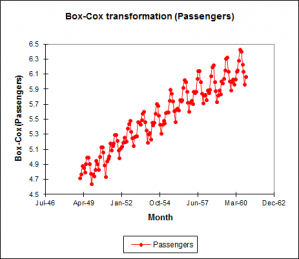# Transformación de series de tiempo

Several time series transformations including Box-Cox can be accessed in Excel using the XLSTAT add-on statistical software.XLSTAT offers four different possibilities for transforming a time series Xt into Yt, (t=1,…,n):

## Box-Cox transform (fixed or optimised)

Box-Cox transformation is used to improve the normality of the time series; the Box-Cox transformation is defined by the following equation:

Yt = [ ( X2t - 1 ) / λ , (Xt > 0, λ ≠ 0 ) or (Xt ≥ 0, λ > 0 ) ; ln( Xt ), (Xt > 0, λ = 0) ]

XLSTAT accepts a fixed value of λ, or it can find the value that maximizes the likelihood of the residuals, the model being a simple linear model with the time as sole explanatory variable.

## Differencing (1-B)d(1-Bs)D

Differencing is to remove trend and seasonalities and to obtain stationarity of the time series. The difference equation writes:

Yt = (1-B)d (1-Bs)D Xt

where d is the order of the first differencing component, s is the period of the seasonal component, D is the order of the seasonal component, and B is the lag operator defined by:

BXt = Xt-1

The values of (d, D, s) can be chosen in a trial and error process, or guessed by looking at the descriptive functions (ACF, PACF). Typical values are (1,1,s), (2,1,s). s is 12 for monthly data with a yearly seasonality, 0 when there is no seasonality.

## Detrending and deseasonalizing

Detrending and deseasonalizing using the classical decomposition model which writes:

Xt = mt + st + εt

where mt is the trend component and st the seasonal component, and εt is a N(0,1) white noise component.

XLSTAT allows fitting this model in two separate and/or successive steps:

### Detrending by polynomial regression

X t = m t + ε t = Σi=0..k aiti + εt

where k is the polynomial degree. The ai parameters are obtained by fitting a linear model to the data. The transformed time series writes:

Y t = ε t = X t - = Σi=0..p aiti

### Desaisonalization by linear model

Xt = st + εt = µ + bi + εt, i = t mod p

where p is the period. The bi parameters are obtained by fitting a linear model to the data. The transformed time series writes: Yt = εt = Xt - µ - bi

Note: there are many other possible transformations. Some of them are available in the transformations tool of XLSTAT see the data transformation section. Linear filters may also be applied. Moving average smoothing methods which are linear filters are available in the Smoothing tool of XLSTAT.### analice sus datos con xlstat

prueba gratuita de 14 días

Incluido en